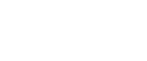## Mathematics Questions and Answers - Form 1 Term 2 Opener Exams 2022

QUESTIONS

1. State the place values of the following digits in the number 201.789.
1. 1(1mk)
2. 8 (1mk)
3. 7(1mk)
2.
1. Write 207,099,099 in words. 2mks
2. What is the total value of 7 in the number in (a) above? 1mk
3. Write in figures: 2mks
Ninety five billion, one hundred and fifty four million, two hundred and twenty three thousand and thirty
4. Determine, without actual division, whether the number 51,257,932 is:
1. divisible by 3 (2mks)
2. divisible by 8 (2mks)
3. divisible by 11 (2mks)
5. Mutai leaves behind 50 hectares of land and sh. 120,000 savings in his will. The land was sold at sh. 80,000 per hectare. If his wife gets sh. 520,000 and the rest is divided equally among his four sons and two daughters, how much money does each child get? 4mks
6. Convert the recurring decimal 0.18 into fraction 3mks
7. Express 900 as a product of its prime factors (2mks)
8. Find their L.C.M and the G.C.D of 24, 60 and 108 using prime factors method and leave your answers as a product of their prime factors. 4mks
9. The L.C.M of three numbers is 24 and their G.C.D is 4. If two of the numbers are 8 and 12, find the other number. 4mks
10. Convert 1¾ into a mixed decimal 2mks
11. Work out the following (4mks)
1. (-5) x (-2) x (-4)
2. (-36) ÷ (-9) × (+2)
12. Three-fifths of work is done on the first day. On the second day, ¾ of the remainder is completed. If third day 7/8 of what remained is done, what fraction of work remains to be done? (3mks)
13. Arrange the following fractions in descending order. (3mks)
7/12, 2/3, ¾, 5/6
14. Use a number line to evaluate: 4mks
1. (+4) + (-6)
2. (-5) – (-5)
15. Evaluate; 3mks
{(1¼ - 3/8) ÷ 2½ + 1¾ ÷ 1¼}
16. Write the 0.12 as fractions in its simplest form. 2mks
17. Write the following in standard form. (2mks)
1. 32890
2. 0.00346
18. Find the squares of the following using mathematical tables.
1. 2594 (2mks)
2. 0.005643 (2mks)
19. Find the square roots of the following numbers using mathematical tables.
1. 5974 (3mks)
2. 0.0000602 (3mks)
20. Simplify: 0.165 x 12.75 (3mks)
0.25 x 0.0075
21. Solve the equation.x+1 + 2x+1  = 9 3mks
2        2## MARKING SCHEME

1.
1. Ones
2. Hundredths
3. Tenths
2.
1. To hundred and seven millio, ninety nine thousand and ninety nine
2. 7,000,000
3. 95 000 000 000
154 000 000
223 000
030
= 95, 154,223,030
4.
1. 5 + 1 + 2 + 5 + 7 + 9 + 3 + 2 = 34
34 isn't a multiple of 3 therefore 51,257,932 is not divisible by 3
2. 51257932
932 is not a multiple of 8 therefore 51257932 is not divisible by 8

3.5 + 2 + 7 + 3 = 17
1 + 5 + 9 + 2 = 17
The difference of the sums of numbers in alternating positions is 0 hence it is divisible by 11
5. Total cash = 50 x 80000 + 120000
= 4120000
Remainder after deducting wife's share = 4120000 - 520000
= 3600000
Children = 3600000
6
= 600000
6. Let r = 0.181818...
10r = 1.81818...
100r = 18.181818....
100r = 18.181818
- r    =    0.181818
99r/99 = 18.0/99
r = 18/99
= 2/11

7.2 x 2 x 3 x 3 x 5 x 5
= 22 x 32 x 52
8. 24 = 23 x 3
60 = 22 x 3 x 52
108 = 22 x 33
LCM = 22 x 33 x 5
GCD = 22 x 3
9. LCM = 23 x 3
GCD= 22
8 = 23
12 = 22 x 3
Other number
22 = 4
OR
LCM x GCD
one of the number
24 x 4 = 12x
8
24 x 4 = 8x
12
LCM x GCD      = 24 x 4 = 4
LCM of number 24
10. 7/4
4√7
4
30
28
20
20
= 1.75
11.
1. = 10 x -4
= -40
2. (-36) x + 2
-9
= +4 x+2
= +8
12. Remainder after day 1
1 - 3/5 = 2/5
Day 2 = ¾ of 2/5
= 3/10
Total = 3/5 + 3/10 = 6 + 3
10
9/10
Remainder = 1 - 9/10
= 1/10
3rd day
7/8 of 1/10
7/80
Total = 9/10 + 7/80= 72 + 7
80
79/80
Remainde= 1/80
13. 7,8,9,10
12
= 10/12 , 9/12 , 8/12 , 7/12
= 5/6 , ¾ , 2/3 , 7/12
14.

1.= -2

2.= 0
15. BODMAS
(5/4 - 3/8) ÷ 2 + 1 ÷ 1
(7/8 ÷ 2)+(1 ÷ 1)
= 7/20 + 7/5 = 7 + 28 = 35/20
= 115/20 = 1
16. 3/25
17.
1. 3.2890 x 104
2. 3.46 x 10-3
18.
1. (2.594 x 103)2
= 2.5942 x 10002
= 6.729 x 1000000
= 6729000
2. (5.643 x 10-3)2
= 5.6432 x (1/1000)2
= 31.84 x 1/1000000
= 0.00003184
19.
1. √59.74 x 102
= √59.74 x √100
= 7.729 x 10
= 77.29
2. √60.2 x 10-6
√60.2 x √1/1000000
7.759 x 1/1000
= 0.007759
20. 0.165 x 12.75 x 1000000
0.25 x 0.0075    1000000
= 165 x 1275 x 10
25 x 75
= 33 x 17 x 2
= 1122
21. (x + 1 + 2x + 1 = 9)2
2           2
x + 1 + 2x + x = 18
3x + 2 = 18
3x = 18 - 2
3x/3 = 16/3
x = 16/3

• ✔ To read offline at any time.
• ✔ To Print at your convenience
• ✔ Share Easily with Friends / Students